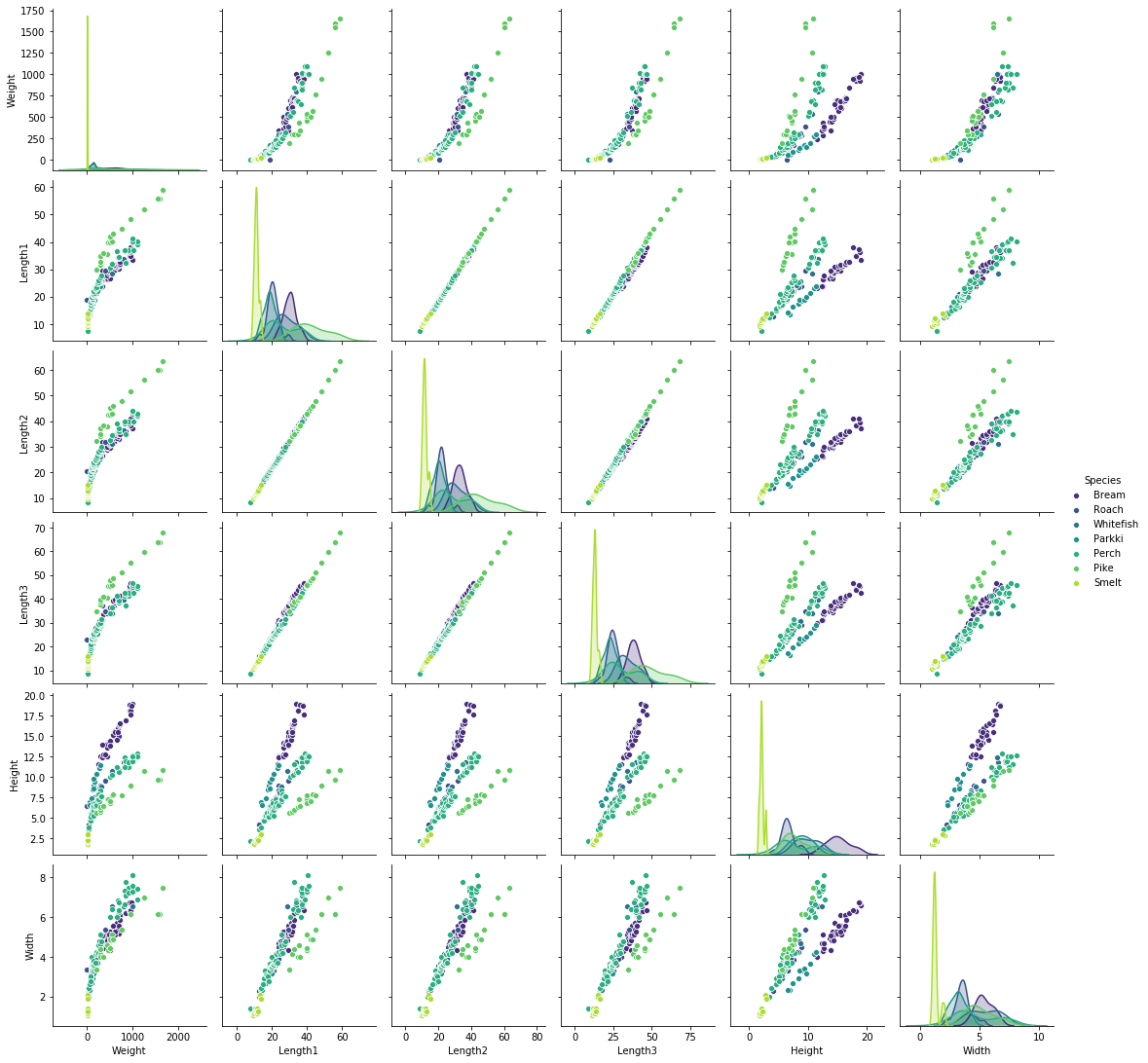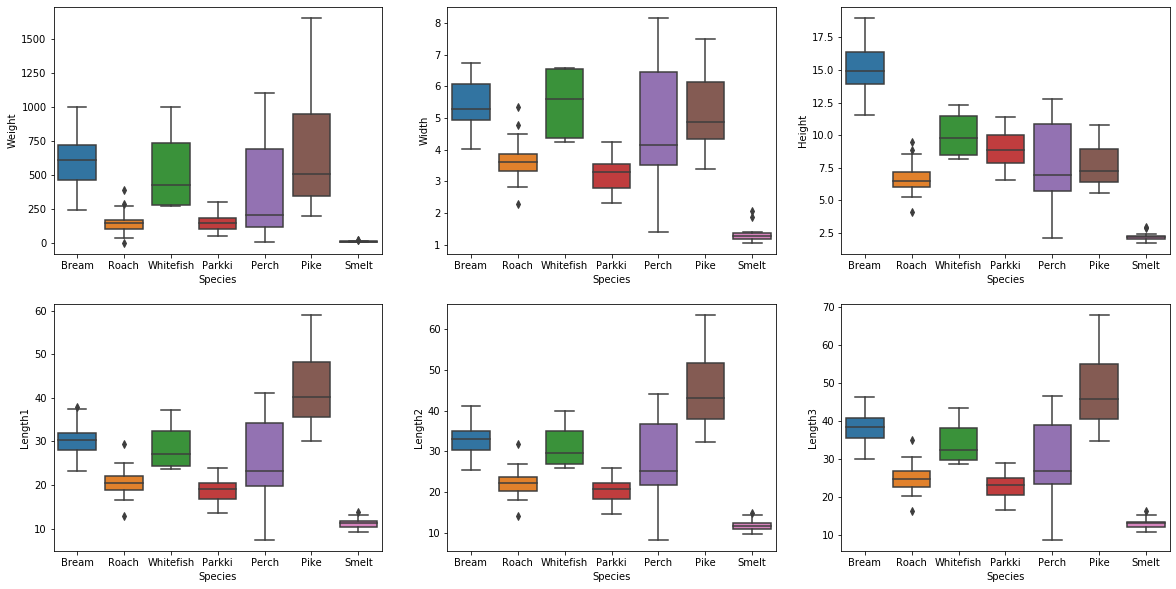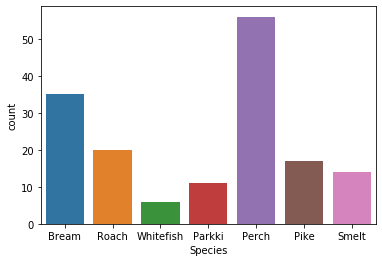# Machine Learning 101: Logistic Regression in PythonLogistic Regression is one of the most basic ML algorithms. While it is a basic algorithm it isn’t always included in ML courses because of its weaknesses.

## Classification vs Regression

Machine Learning essentially deals with two kinds of problems:

• Classification: predicting a class, for example whether a user is male or female (the two classes) given their history of purchased items.
• Regression: predicting a value, for example the price (the value) of a used car given the model, the age, the kilometers on the odometer.

It is important to remember that Machine Learning is no magic, ML algorithms are still algorithms: multiple inputs, one output. The most important difference between a traditional algorithm and an ML one is the “experience” the ML algorithm gains during the training phase.

In Classification problems the algorithm tries to predict the class the entry will fall into, it may be two classes (such as the example above, male versus female) or more than two classes. The former is often called Binary Classification the latter is referred to as Multiclass Classification.

In Regression there is no class to predict, instead there is a scale and the algorithm tries to predict the value on that scale. In the example above the price is the sought value.

## Logistic Regression in Python

Explaining what Logistic Regression is without delving too much into mathematics is actually quite difficult. Know that this technique is pretty similar to Linear Regression when it comes to method, but the underlying function is not a line, it instead is the logistic function (often called sigmoid function):

$$f(x) = \frac{L}{1 + e^{-k(x-x_0)}}$$

## Logistic regression using fish (classification problem)

The following notebook uses the Fish market dataset available here, it is free and released under the GPL2 license. This dataset includes a number of species of fish and for each fish some measurements such as weight and height.

### (Basic) Explanatory Data Analysis

Every good ML algorithm should start with an in-depth Explanatory Data Analysis (EDA). In the EDA you should always try to explore the data as much as possible, through exploration it is possible to infer basic features of the data, from those basic inferences you can start developing a basic intuition. From there you can start formulating hypotheses and implement the algorithm you see fit.

As the purpose of this notebook is to illustrate Logistic Regression applied to a Classification Problem, the performed EDA will outline just the basic features of the dataset.

Firstly, let's import basic utilities:

In :
%matplotlib inline
import pandas as pd
import numpy as np
import matplotlib.pyplot as plt
import seaborn as sns

np.random.seed(101) # This is needed so that if you run this notebook again you will get the same results


Let's now read the csv file (keep in mind it should be in the same folder as the notebook!):

In :
df = pd.read_csv('Fish.csv')


Let's take a look at the first rows of the dataset. It is important to do this in order to get a basic understanding.

In :
df.head()

Out:
Species Weight Length1 Length2 Length3 Height Width
0 Bream 242.0 23.2 25.4 30.0 11.5200 4.0200
1 Bream 290.0 24.0 26.3 31.2 12.4800 4.3056
2 Bream 340.0 23.9 26.5 31.1 12.3778 4.6961
3 Bream 363.0 26.3 29.0 33.5 12.7300 4.4555
4 Bream 430.0 26.5 29.0 34.0 12.4440 5.1340

Now let's take a closer look to the dataset to get important statistical indicators such as the mean and standard deviation

In :
df.describe()

Out:
Weight Length1 Length2 Length3 Height Width
count 159.000000 159.000000 159.000000 159.000000 159.000000 159.000000
mean 398.326415 26.247170 28.415723 31.227044 8.970994 4.417486
std 357.978317 9.996441 10.716328 11.610246 4.286208 1.685804
min 0.000000 7.500000 8.400000 8.800000 1.728400 1.047600
25% 120.000000 19.050000 21.000000 23.150000 5.944800 3.385650
50% 273.000000 25.200000 27.300000 29.400000 7.786000 4.248500
75% 650.000000 32.700000 35.500000 39.650000 12.365900 5.584500
max 1650.000000 59.000000 63.400000 68.000000 18.957000 8.142000

Let's now plot each numerical feature against each other, in order to get a clear distinction use a palette with high contrast (Viridis) and use the species as color.

In :
sns.pairplot(df, hue='Species', palette='viridis')

Out:
<seaborn.axisgrid.PairGrid at 0x1e5dff1c748>As you can see there is quite a strong pattern between: Length1 and Length2, Length2 and Length3, Length3 and Length1.

In the next step we will try to predict whether the fish is a Perch or a Bream, so let's get a better look at each feature for each species.

In :
fig, axes = plt.subplots(ncols=3, nrows=2, figsize=(20, 10), sharey=False)
sns.boxplot(data=df, y='Weight', x='Species', ax=axes)
sns.boxplot(data=df, y='Width', x='Species', ax=axes)
sns.boxplot(data=df, y='Height', x='Species', ax=axes)
sns.boxplot(data=df, y='Length1', x='Species', ax=axes)
sns.boxplot(data=df, y='Length2', x='Species', ax=axes)
sns.boxplot(data=df, y='Length3', x='Species', ax=axes)

Out:
<matplotlib.axes._subplots.AxesSubplot at 0x1e5e1870c88>As you can see Length1, Length2 and Length3 follow essentially the same pattern across each species (hence they contain almost the same information). On the other hand each species has a set of Width, Height and Weight.

As the dataset is quite small it may be worth knowing how many observations there are for each fish species:

In :
sns.countplot(data=df, x='Species')

Out:
<matplotlib.axes._subplots.AxesSubplot at 0x1e5e1cbea88>### Logistic regression (binary classification): Let's predict whether the fish is a Perch or a Bream

In this step a Logistic Regression model to predict the fish species will be created. In order to make it simpler for the model to make predictions we will restrict the domain of species to Perch and Bream species. The model will be able to tell a Perch apart from a Bream, but any other fish it will have no clue.

The following lines allow us to create a X variable containing all the features except "Species", and a y variable containing just the species. Both variables will only include observations about Perch and Bream species.

In :
X = df[(df['Species'] == 'Perch') | (df['Species'] == 'Bream')].drop('Species', axis=1)
y = df[(df['Species'] == 'Perch') | (df['Species'] == 'Bream')]['Species'].replace(['Bream', 'Perch'], [0, 1])


Split the dataset in two parts: train and test. This is needed to calculate the accuracy (and many other metrics) of the model. We will use the train part during the training, and the test part during the evaluation. The model will not see the test part during its training.

In :
from sklearn.model_selection import train_test_split
X_train, X_test, y_train, y_test = train_test_split(X, y, test_size = 0.33, random_state=101)


Without delving too much into mathematics, using Logistic Regression involves using a method to solve the equations. In order to make said method converge (find the solution) faster, data is usually standardized. You can standardize any dataset by doing:

$$\mathcal{z}=\frac{x-\mu}{\sigma}$$

Where $\mu$ is the mean average and $\sigma$ is the standard deviation of each particular column. You can work the math for each column and assign them using pandas features, or you can leverage sklearn StandardScaler. The StandardScaler will first need to be fitted (calculate $\mu$ and $\sigma$). Then the scaler will be used to scale X_train and X_test. You don't need to scale the y variable (it also only has boolean values in it (0, 1). Another thing you don't want to do is fit the scaler on train and test data, by doing this you will throw off the parameters and "leak" information (you will inherently tell bits of how the X_test looks like). Once the scaler has been fitted to the data, the data can be transformed using the formula above.

In :
from sklearn.preprocessing import StandardScaler

scaler = StandardScaler()
scaler = scaler.fit(X_train)
X_train = scaler.transform(X_train)
X_test = scaler.transform(X_test)


Import the model and instantiate it:

In :
from sklearn.linear_model import LogisticRegression

lr = LogisticRegression()


Now let's train the model:

In :
lr.fit(X_train, y_train)

Out:
LogisticRegression(C=1.0, class_weight=None, dual=False, fit_intercept=True,
intercept_scaling=1, l1_ratio=None, max_iter=100,
multi_class='auto', n_jobs=None, penalty='l2',
random_state=None, solver='lbfgs', tol=0.0001, verbose=0,
warm_start=False)

In order to review our model we will use two handy functions: the classification report (which sums up useful statistics, and the confusion matrix.

In :
from sklearn.metrics import classification_report, confusion_matrix

In :
print(classification_report(y_test, lr.predict(X_test)))

              precision    recall  f1-score   support

0       1.00      1.00      1.00        14
1       1.00      1.00      1.00        17

accuracy                           1.00        31
macro avg       1.00      1.00      1.00        31
weighted avg       1.00      1.00      1.00        31



While discussing about the matrics deserves a whole article by itself, it is easy to observe the model is a perfect fit, achieving 100% precision and recall. The "support" column tells you how many observation support the class (0, 1). Let's now take a look at the confusion matrix:

In :
print(confusion_matrix(y_test, lr.predict(X_test)))

[[14  0]
[ 0 17]]


While the classification report allows for a quick review of the model performance, the confusion matrix takes a bit more to be understood.

• The upper left number represents true positives (they are 1, classified as 1)
• The upper right number represents false positives (they are 0, classified as 1)
• The lower left number represents false negatives (they are 1, classified as 0)
• The lower right number represents true negatives (they are 0, classified as 0)

#### Conclusion: It's prefect!

Although the model can only predict whether the fish is a Perch or a Bream, it seems to be working perfectly, but is it really? First of all as you can saw during the EDA, Breams and Perches are essentially different fishes. You should also keep in mind the input of the model (weight, length1, length2, length3, width and height) is essentially shared among ALL fishes in the world. Imagine you find a fish whose features are essentially the same of a Bream but it is not a Bream. Well, the model would classify it as a Bream, making an error. Since the model trained on Bream and Perch species only (albeit with a very small dataset), you should be sure you're trying to classify one of the two.

### Logistic Regression (multiclass problem): Let's predict the species

This problem closely resembles the last one, yet we're now trying to predict the species. There are seven species in this dataset and there aren't many observations for some of them.

In :
X = df.drop(['Species'], axis=1)
y = df['Species']

In :
from sklearn.model_selection import train_test_split
X_train, X_test, y_train, y_test = train_test_split(X, y, test_size = 0.33, random_state=101)

In :
from sklearn.preprocessing import StandardScaler

scaler = StandardScaler()
scaler = scaler.fit(X_train)
X_train = scaler.transform(X_train)
X_test = scaler.transform(X_test)

In :
from sklearn.linear_model import LogisticRegression

lr = LogisticRegression()

In :
lr.fit(X_train, y_train)

Out:
LogisticRegression(C=1.0, class_weight=None, dual=False, fit_intercept=True,
intercept_scaling=1, l1_ratio=None, max_iter=100,
multi_class='auto', n_jobs=None, penalty='l2',
random_state=None, solver='lbfgs', tol=0.0001, verbose=0,
warm_start=False)

Let's now evaluate the model:

In :
from sklearn.metrics import classification_report

In :
print(classification_report(y_test, lr.predict(X_test), zero_division=0))

              precision    recall  f1-score   support

Bream       0.90      1.00      0.95         9
Parkki       1.00      0.67      0.80         6
Perch       0.71      1.00      0.83        20
Pike       1.00      1.00      1.00         5
Roach       0.00      0.00      0.00         7
Smelt       1.00      1.00      1.00         5
Whitefish       0.00      0.00      0.00         1

accuracy                           0.81        53
macro avg       0.66      0.67      0.65        53
weighted avg       0.72      0.81      0.75        53



Where's the confusion matrix? If you print the confusion matrix for a multiclass problem you will see it is actually quite difficult to interpret its results, stick to the classification report for easier-to-understand values. For the curious ones:

In :
print(confusion_matrix(y_test, lr.predict(X_test)))

[[ 9  0  0  0  0  0  0]
[ 1  4  0  0  1  0  0]
[ 0  0 20  0  0  0  0]
[ 0  0  0  5  0  0  0]
[ 0  0  7  0  0  0  0]
[ 0  0  0  0  0  5  0]
[ 0  0  1  0  0  0  0]]


### Conclusion: Not so accurate now?

As the previous model achieved 100% accuracy and recall, the second one achieved an overall accuracy of 0.81. As you can see as the number of species grow and the features overlap, the model struggles to distinguish between fish species with some species such as Roach and Whitefish being mispredicted all of the times. Also Perches and Breams that were previously classified perfectly are now not as certain as before. The size of the dataset, the presence of outliers, the weaknesses of the model all show up together in the last report. Know that Logistic Regression may not be the best algorithm to use in this case.

## Conclusion

You now know what Logistic Regression is about, how to use it and hopefully when you shouldn’t use it (mutliclass problems).

Image courtesy of mark | marksei

The following two tabs change content below.#### mark

The IT guy with a slight look of boredom in his eyes. Freelancer. Current interests: Kubernetes, Tensorflow, shiny new things.#### You may also like...

This site uses Akismet to reduce spam. Learn how your comment data is processed.